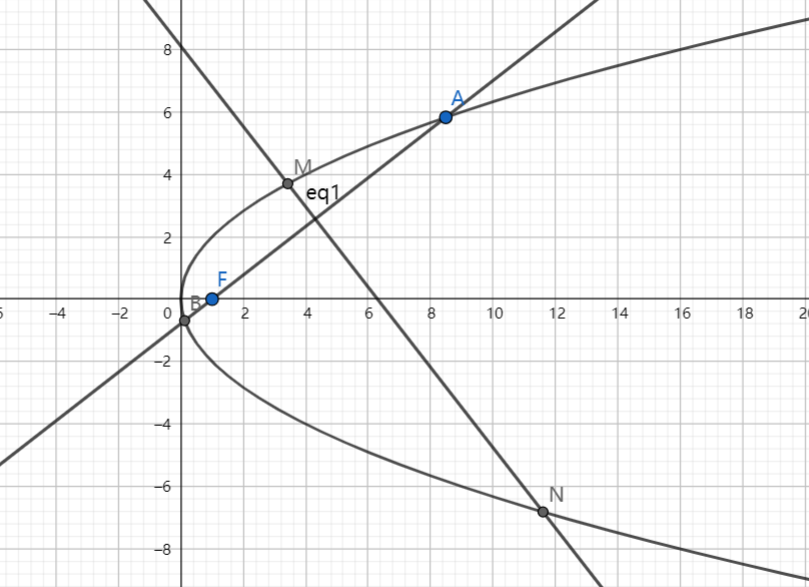# SAT1000 - P844

Geometry Level pendingAs shown above, $F(1,0)$ is the focus of the parabola $C: y^2=4x$, line $l: y=k(x-1)$ intersects with $C$ at point $A,B$, and the perpendicular bisector of $AB$ intersects with $C$ at point $M,N$.

If $A,M,B,N$ are on the same circle, then find the value of $\lfloor 1000|k| \rfloor$.

Have a look at my problem set: SAT 1000 problems

×